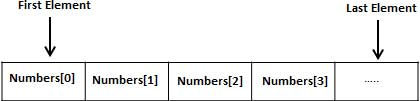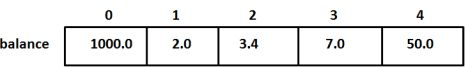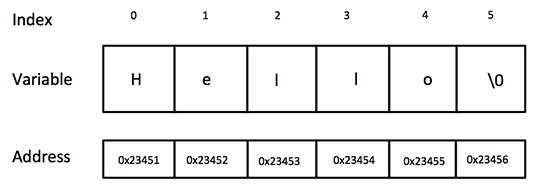## 8240819346

##### EMAIL:
###### info@mcatutorials.com
Enroll now!
 Name Cources BTECH MCA MSC BCA BSC Others -- Choose Your Subject -- Computer Organization C Language Data Structure Digital Electronics Object Oriented Programming Discrete Mathematics Graph Theory Operating Systems Software Engineering Computer Graphics Database Management System Operation Research Computer Networking Image Processing Internet Technologies Micro Processor Artificial Intelligence Soft Computing E-Commerce & ERP C ++ Language C Programming C Data Structure Using C, C ++ Sql & PL/Sql Visual Basic Unix and Shell programming Java J2EE Oracle 9i 8085 Programming Android Application Development i-phone Application Development JAVA Swing based applications PHP, MySql, Ajax ASP.net Visual Basic Client Server Applications Email Phone# Array

C programming language provides a data structure called the array, which can store a fixed-size sequential collection of elements of the same type. An array is used to store a collection of data, but it is often more useful to think of an array as a collection of variables of the same type.

Instead of declaring individual variables, such as number0, number1, ..., and number99, you declare one array variable such as numbers and use numbers, numbers, and ..., numbers to represent individual variables. A specific element in an array is accessed by an index.

All arrays consist of contiguous memory locations. The lowest address corresponds to the first element and the highest address to the last element.## Declaring Arrays

To declare an array in C, a programmer specifies the type of the elements and the number of elements required by an array as follows:

```type arrayName [ arraySize ];
```

This is called a single-dimensional array. The arraySize must be an integer constant greater than zero and type can be any valid C data type. For example, to declare a 10-element array called balance of type double, use this statement:

```double balance;
```

Now balance is avariable array which is sufficient to hold upto 10 double numbers.

## Initializing Arrays

You can initialize array in C either one by one or using a single statement as follows:

```double balance = {1000.0, 2.0, 3.4, 7.0, 50.0};
```

The number of values between braces { } can not be larger than the number of elements that we declare for the array between square brackets [ ].

If you omit the size of the array, an array just big enough to hold the initialization is created. Therefore, if you write:

```double balance[] = {1000.0, 2.0, 3.4, 7.0, 50.0};
```

You will create exactly the same array as you did in the previous example. Following is an example to assign a single element of the array:

```balance = 50.0;
```

The above statement assigns element number 5th in the array with a value of 50.0. All arrays have 0 as the index of their first element which is also called base index and last index of an array will be total size of the array minus 1. Following is the pictorial representation of the same array we discussed above:## Accessing Array Elements

An element is accessed by indexing the array name. This is done by placing the index of the element within square brackets after the name of the array. For example:

```double salary = balance;
```

The above statement will take 10th element from the array and assign the value to salary variable. Following is an example which will use all the above mentioned three concepts viz. declaration, assignment and accessing arrays:

```#include <stdio.h>

int main ()
{
int n[ 10 ]; /* n is an array of 10 integers */
int i,j;

/* initialize elements of array n to 0 */
for ( i = 0; i < 10; i++ )
{
n[ i ] = i + 100; /* set element at location i to i + 100 */
}

/* output each array element's value */
for (j = 0; j < 10; j++ )
{
printf("Element[%d] = %d\n", j, n[j] );
}

return 0;
}
```

When the above code is compiled and executed, it produces the following result:

```Element = 100
Element = 101
Element = 102
Element = 103
Element = 104
Element = 105
Element = 106
Element = 107
Element = 108
Element = 109
```

## C Arrays in Detail

Arrays are important to C and should need lots of more details. There are following few important concepts related to array which should be clear to a C programmer:

ConceptDescription
Multi-dimensional arraysC supports multidimensional arrays. The simplest form of the multidimensional array is the two-dimensional array.
Passing arrays to functionsYou can pass to the function a pointer to an array by specifying the array's name without an index.
Return array from a functionC allows a function to return an array.
Pointer to an arrayYou can generate a pointer to the first element of an array by simply specifying the array name, without any index.

The string in C programming language is actually a one-dimensional array of characters which is terminated by a null character '\0'. Thus a null-terminated string contains the characters that comprise the string followed by a null.

The following declaration and initialization create a string consisting of the word "Hello". To hold the null character at the end of the array, the size of the character array containing the string is one more than the number of characters in the word "Hello."

```char greeting = {'H', 'e', 'l', 'l', 'o', '\0'};
```

If you follow the rule of array initialization then you can write the above statement as follows:

```char greeting[] = "Hello";
```

Following is the memory presentation of above defined string in C/C++:Actually, you do not place the null character at the end of a string constant. The C compiler automatically places the '\0' at the end of the string when it initializes the array. Let us try to print above mentioned string:

```#include <stdio.h>

int main ()
{
char greeting = {'H', 'e', 'l', 'l', 'o', '\0'};

printf("Greeting message: %s\n", greeting );

return 0;
}
```

When the above code is compiled and executed, it produces result something as follows:

```Greeting message: Hello
```

C supports a wide range of functions that manipulate null-terminated strings:

S.N.Function & Purpose
1strcpy(s1, s2);
Copies string s2 into string s1.
2strcat(s1, s2);
Concatenates string s2 onto the end of string s1.
3strlen(s1);
Returns the length of string s1.
4strcmp(s1, s2);
Returns 0 if s1 and s2 are the same; less than 0 if s1<s2; greater than 0 if s1>s2.
5strchr(s1, ch);
Returns a pointer to the first occurrence of character ch in string s1.
6strstr(s1, s2);
Returns a pointer to the first occurrence of string s2 in string s1.

Following example makes use of few of the above-mentioned functions:

```#include <stdio.h>
#include <string.h>

int main ()
{
char str1 = "Hello";
char str2 = "World";
char str3;
int  len ;

/* copy str1 into str3 */
strcpy(str3, str1);
printf("strcpy( str3, str1) :  %s\n", str3 );

/* concatenates str1 and str2 */
strcat( str1, str2);
printf("strcat( str1, str2):   %s\n", str1 );

/* total lenghth of str1 after concatenation */
len = strlen(str1);
printf("strlen(str1) :  %d\n", len );

return 0;
}
```

When the above code is compiled and executed, it produces result something as follows:

```strcpy( str3, str1) :  Hello
strcat( str1, str2):   HelloWorld
strlen(str1) :  10
```

You can find a complete list of c string related functions in C Standard Library.